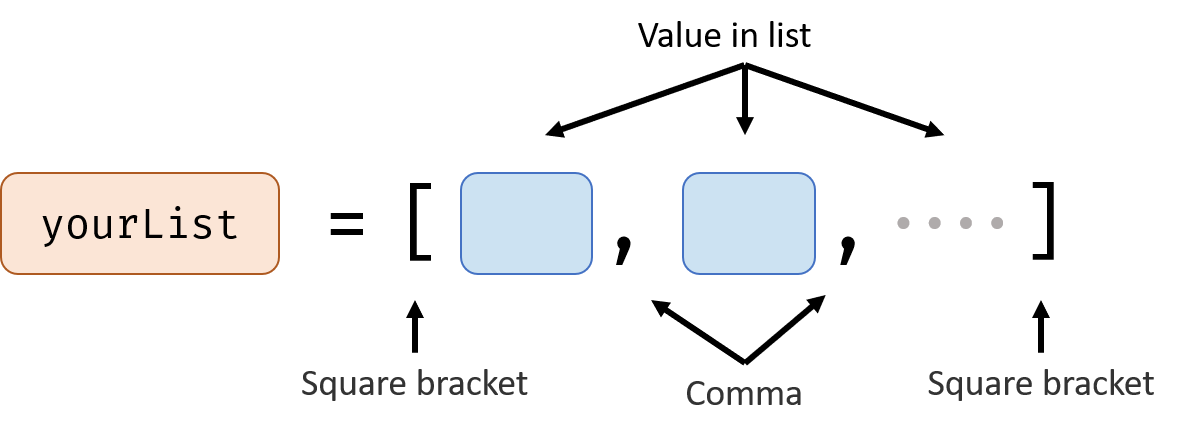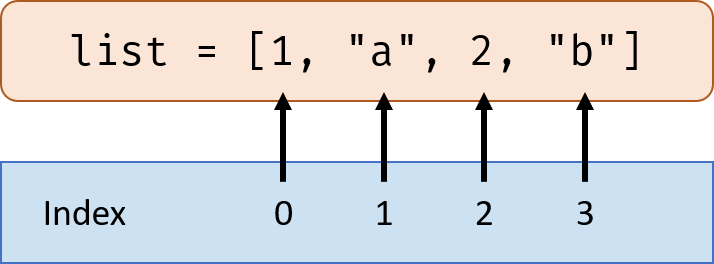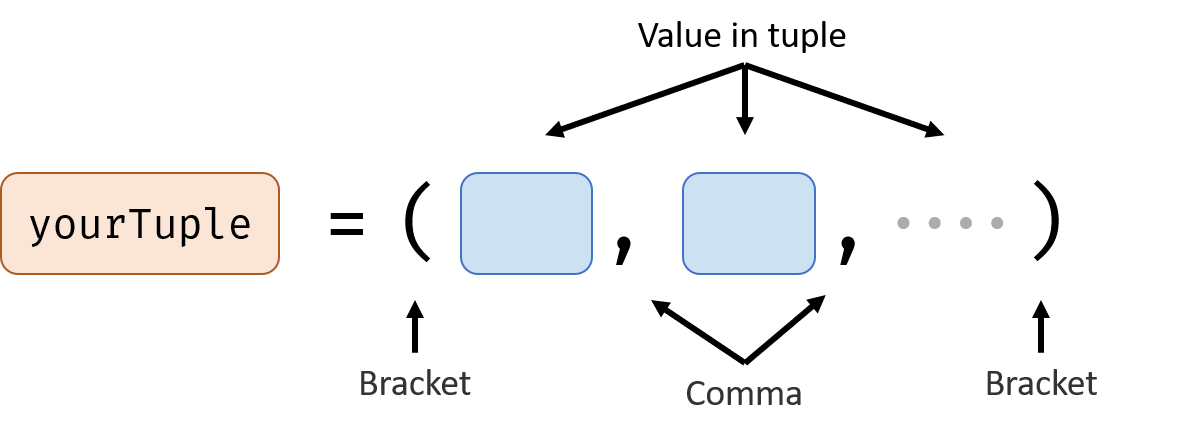# Lists

List is a type of value, a list contains many values, you can add or delete an item from the list.

## How to Represent a ListFlowing is the example of two lists, the first list is filled with numbers, the second list is filled with strings.

You can put values that have different types to an list, for example:

## Index of the List

Now we have a list, but how to get a specific value from the list? For example, how to get the second value in the list?

Use Index.In the list list = [1,"a",2,"b"], the index for 1 is 0, index for "a" is 1, index increases after each item in the list. Note: Remember the index for the first item in the list is 0, not 1.

We can get a value from a list using index like this:

list means the item in the list which have an index of 1. The 1 in the square bracket represents the index.

We can also get a part from the list like this:

list[3:6] means get the part of the list starting from index 3 to index 6. The number before colon is the starting index, the number after colon is the ending index.

## Add, Update and Remove Items from the List

If you want to add one value to the end of the list, use list.append(item)

If you want to insert a value to the middle of the list, use list.insert(index, item)

Example:

### Update

If you want to change the value of one item in the list, use list[index] = newValue

Example:

### Remove

If you know the index and want to remove it from the list, use list.pop(index)

If you know the value and want to remove it from the list, use list.remove(value)

Example:

## Get Information About the List

Length of the list: len(list)

If the list is all number or all string, you can use max(list) and min(list) to get the max and min value in the list.

Example:

## List Operators

Yes, you can add 2 lists XD

# Tuple

A tuple is almost same as a list, except you can’t change the value of it after you define it.

## How to Represent a TupleFor example:

## Aspects That Different From List

Because of tuple is not changeable after defining it, so you can’t add or update or remove an item from the tuple.

## Aspects that same as List

You can get value from a tuple, get length, max value and min value from a tuple.

Because operator doesn’t change the item in a tuple, so you can use operators with tuple.